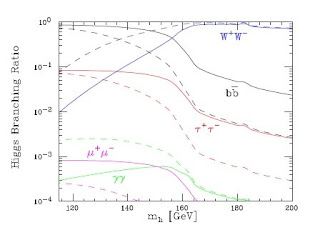## Tuesday, 29 April 2008

### Eastern Flavor

Oh, that was really long time no see...I guess both my fans have become uneasy. Time was scarce earlier this month, while the talks I heard were not so attractive as to trigger me. Now that I'm on holiday on California beach I can finally catch up on blogging.

Last Thursday Oleg Lebedev discussed his recent paper about Higgs-dependent Yukawacouplings. In the Standard Model, the fermion masses originate from Yukawa interactions between the Higgs field and two fermions, $Y \psi_1 H \psi_2$. Renormalizability implies that $Y$ has to be a constant. The simple idea from Oleg's paper is to drop that assumption and see what happens when the Yukawa couplings depend on the Higgs field. Quite generally, one can write $Y = Y^{(0)} + Y^{(2)} (H^\dagger H/M^2) + ...$, where $M$ is some new physics scale - TeV, or higher. Such a structure can readily arise from integrating out heavy states with masses of order M, one example being heavy vector-like fermions coupled to the light fermions via standard Yukawa interactions.

In a general situation, the Higgs-dependent Yukawa couplings are everything but spectacular. They just show up as small deviations of the Higgs couplings to the fermions - something rather hard to pinpoint at the LHC. Things become interesting when the first terms in the expansion vanish for some reason. Except that it's fun, it could shed some light on the fermion mass puzzle, in a similar spirit to the Frogatt-Nielsen mechanism. Those fermions whose Yukawa couplings lack the first $n$ terms in the expansion will have their masses suppressed by $(v^2/M^2)^n$. By engineering the integers $n$, one can explain the hierachical fermion masses in the Standard Model without introducing small parameters. For example, the bottom quark can be assigned $n = 1$, so that its Yukawa coupling would be $Y_b^{(2)} (H^\dagger H/M^2)+ ...$. If $Y_b^{(2)} \sim 1$, matching to the observed bottom quark mass fixes the new physics scale $M$ to be 1-2 TeV. For lighter quarks one can assign larger $n$, so as to obtain small masses with order one Yukawa couplings.

One consequence of such a scenario is that the Higgs physics at the LHC changes dramatically(for worse, as usual). The couplings of the Higgs boson to the quarks are enhanced by $(1 + 2n)$ with respect to the Standard Model value. Therefore the Higgs decay rates change by $(1+2n)^2$. In the plot shown here, the branching ratios are compared to the Standard Model ones (dashed). As long as the Higgs boson is heavy enough to decay into W or Z, the modifications are not very much pronounced. If the Higgs is fairly light, however, the swampy decay to the bottom quark is boosted by a factor of 9 so that the golden Higgs-to-two-photons decay has a much smaller branching ratio. That's another sleazy attempt to prevent a quick and easy Nobel prize for the LHC.

The other side of the coin is that the modified Higgs couplings induce flavor changing neutral currents (FCNC). The lack of tree-level FCNCs in the Standard Model relies on the fact that the Higgs couplings are aligned with the Yukawa couplings. Once $n$ is different for different quark flavors, the alignment is gone, and the Higgs boson mediates FCNCs. Therefore, the model like that may easily run into trouble with experiment. Oleg argued that he can find patterns of $n$'s for which FCNCs are suppressed. Nevertheless, CP violation in the kaon mixing is ridiculously well measured and some fine-tuning is necessary to pass that test. If you need a reason not to believe in the modified Yukawa couplings, that's probably the best one.